# Ray Tracer

## Project Description

For my final project, I created a ray tracer in C++ using GLM, to support the rendering of spheres and planes. My ray tracer implements

## Process

The first step in creating my ray tracer was to implement ray intersection detection with a sphere given the implicit equation of a sphere: (x - xc)² + (y - yc)² + (z - zc)² + R² = 0. The rays used in this equation are viewing rays computed by projecting outwards from a camera located at the origin in the direction of a screen located at a z-value of -1;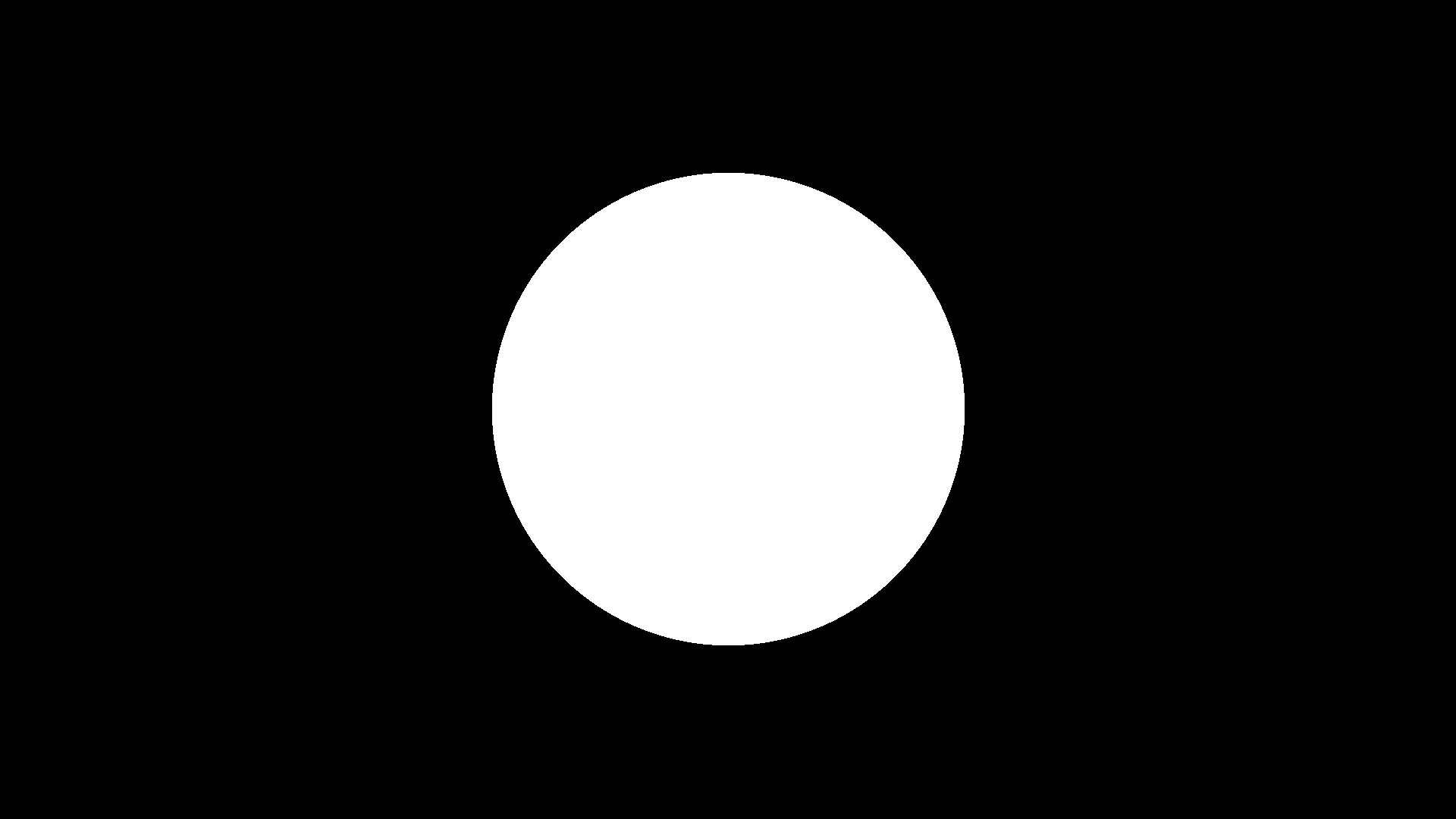Bla: The shape of the sphere created through ray sphere intersections

After the ray-sphere intersection was implemented, I computed the normals of the sphere based on the points of intersection in relation to the center and radius of the sphere.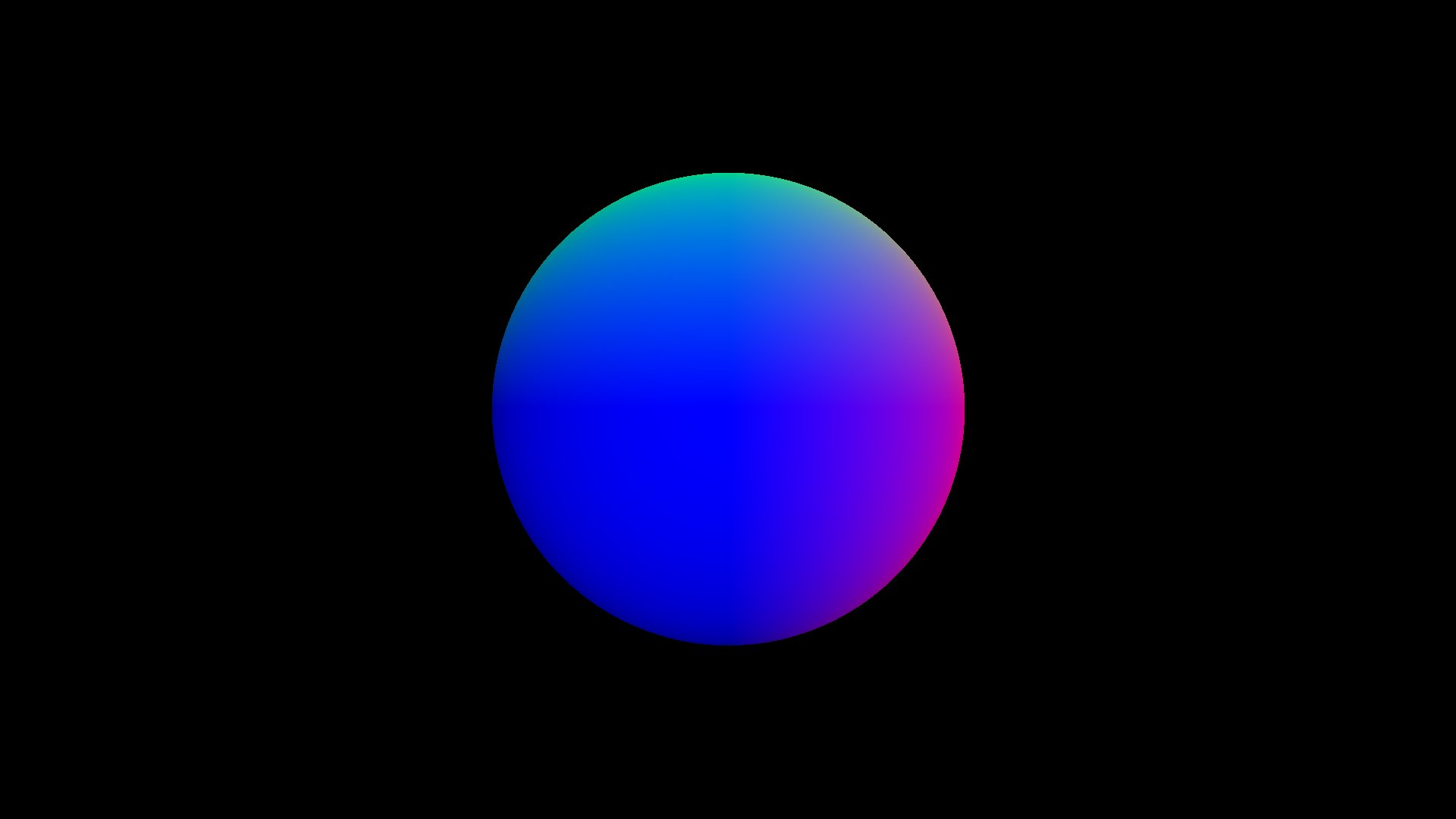Figure 2: The normals of the sphere mapped to RGB values

After computing the normals for the sphere, I implemented a depth buffer to allow multiple spheres to be displayed correctly on the screen.Figure 3: Three normal mapped spheres with appropriate layering

The next step in my ray tracer was to implement the Phong Lighting model: Reflected Color = Diffuse + Specular + Ambient. This step included adding a light source to the scene, and associating material properties with each sphere.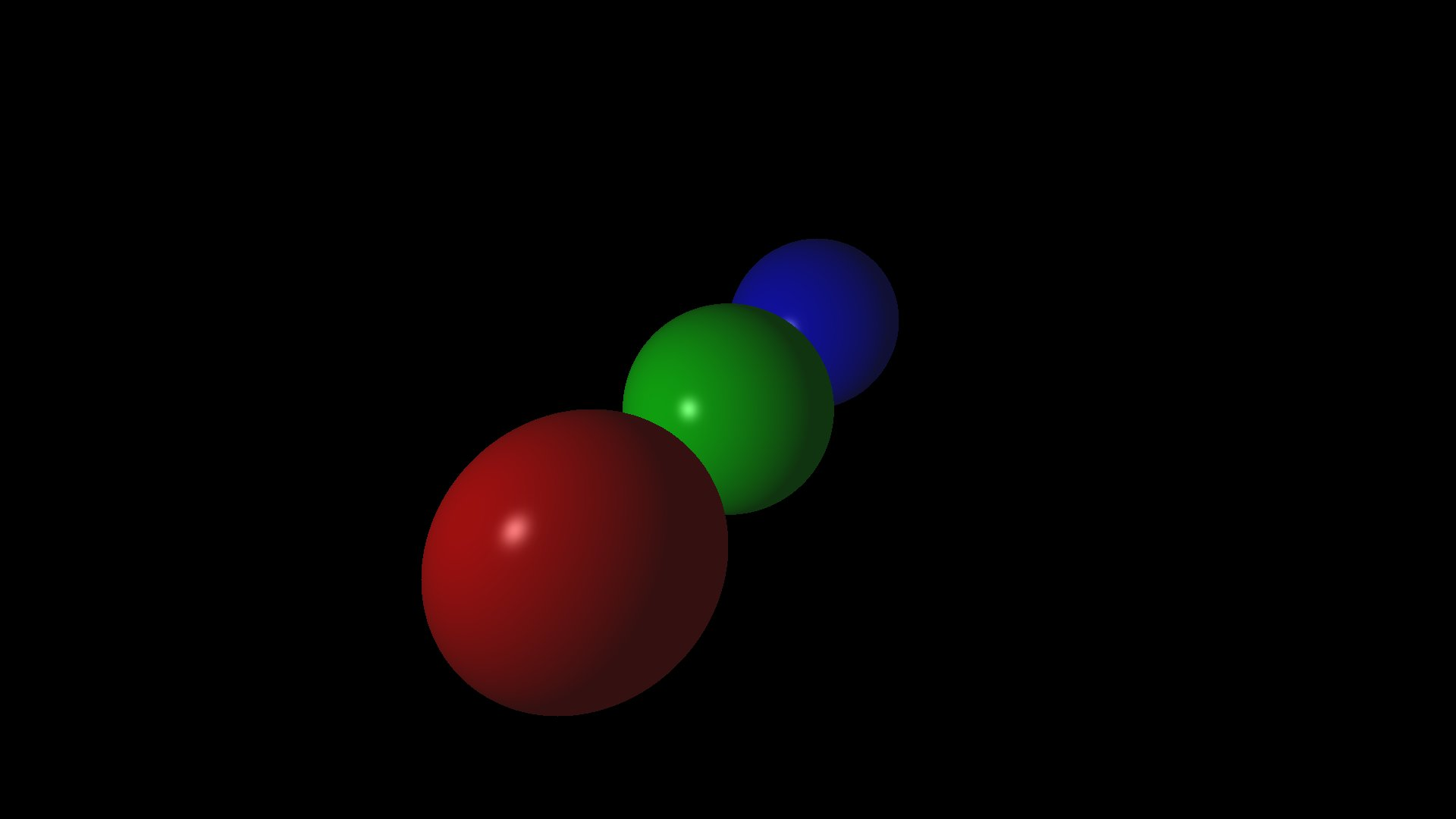Figure 4: Phong Lighting of the spheres

After implementing Phong Lighting, the next step in my ray tracing program was to include shadows. To include shadows, I compute a shadow ray for each point that is the closest intersection for a viewing ray originated at the camera. The shadow ray is computed from the intersection point towards the light source. Using the same process of ray intersection used earlier, the shadow ray is used to determine whether or not there is an intersection with an object between the point and the light source. If there is not an intersection, the point is not in shadow, and receives the full color of the Phong Lighting model. If there is an intersection, the point is in the shadow of another object, and only the ambient color of that point is applied.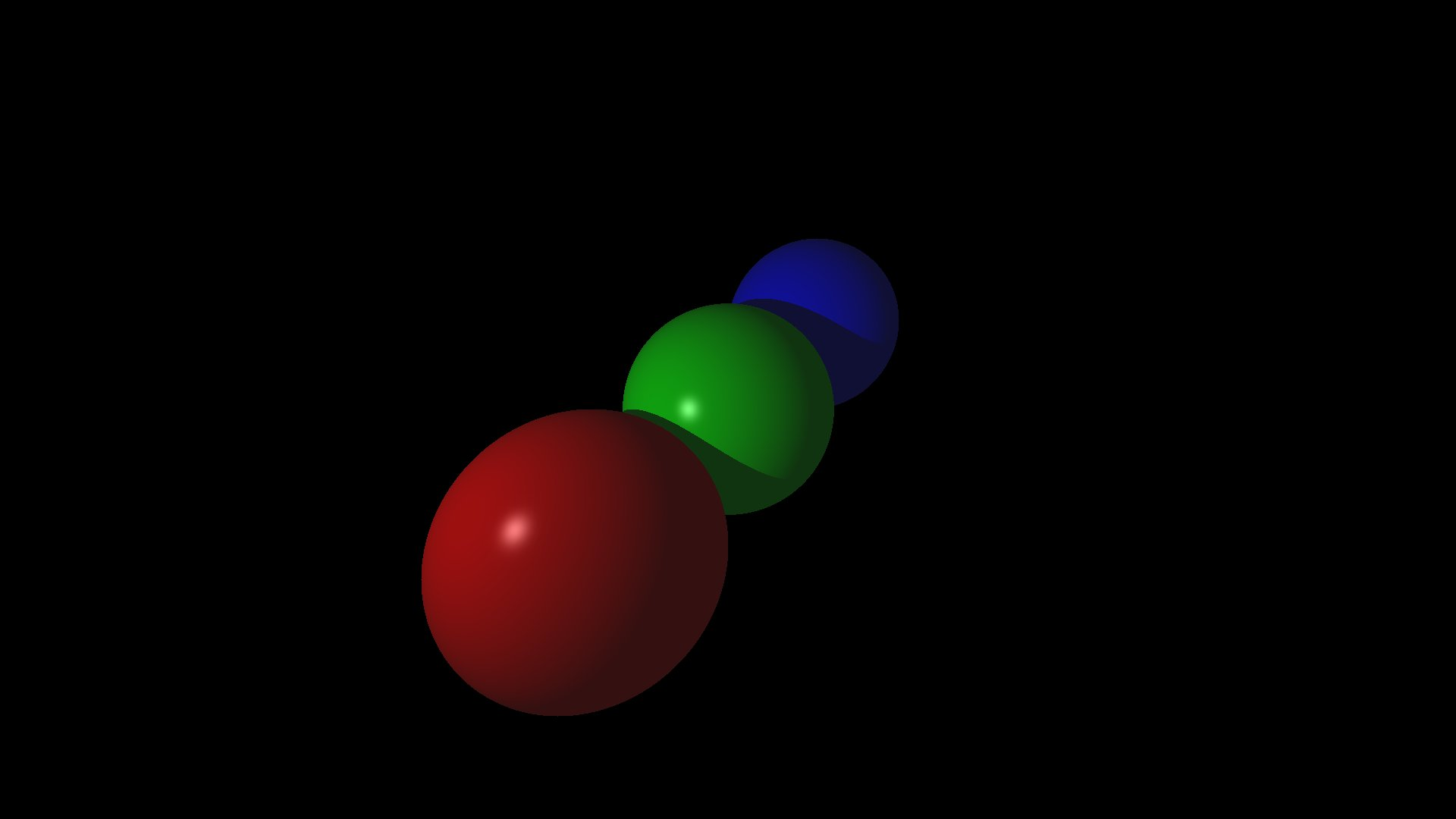Figure 5: Inclusion of shadows on the Phong lit spheres

After implementing shadows, I added support for planes in addition to spheres. A plane's normal is the same at all points on the plane, so the normal does not have to be computed for each individual point of intersection, but rather, may be stored as a property of the plane. Implementing ray intersection for planes allowed the use of the same lighting and shadowing methods used for spheres.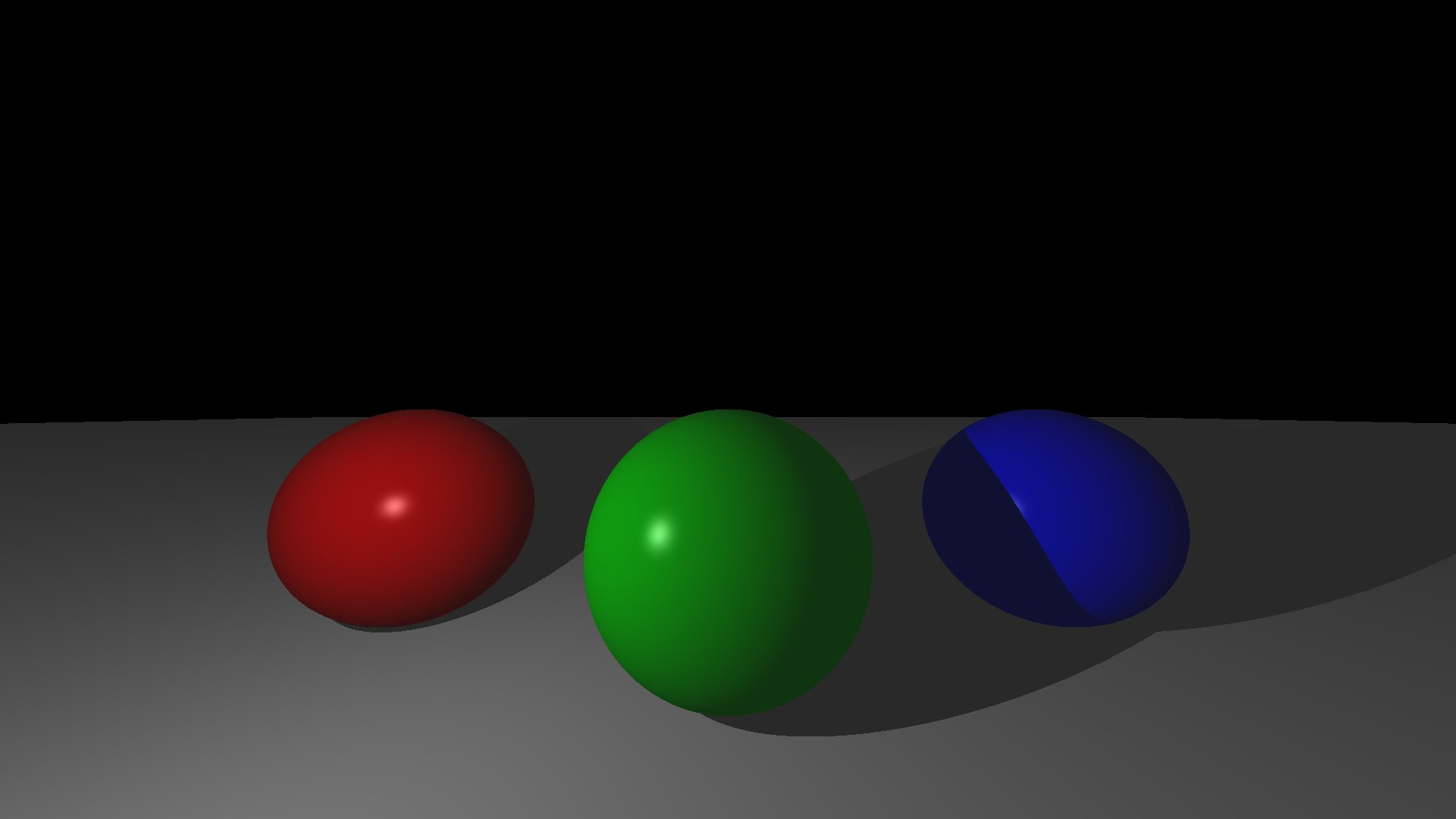Figure 6: Three Phong lit spheres on a Phong lit plane

The final step in implementing my ray tracer was to include reflections. Reflection rays are computed per intersected point, just like shadows. However, differently from shadows, reflections are implemented recursively, to allow a reflection ray to bounce off of several objects. A reflection ray is computed similarly to the reflection vector used in the Phong Lighting model. The reflection ray is then projected from the intersected point, and behaves similarly to the primary viewing ray projected from the camera. If the reflection vector intersects an object, the Phong Lighting value of that intersected object is returned to the original point. To prevent infinite recursion of reflection vectors, I included a maximum reflection depth, which can be easily changed to allow for deeper levels of reflection.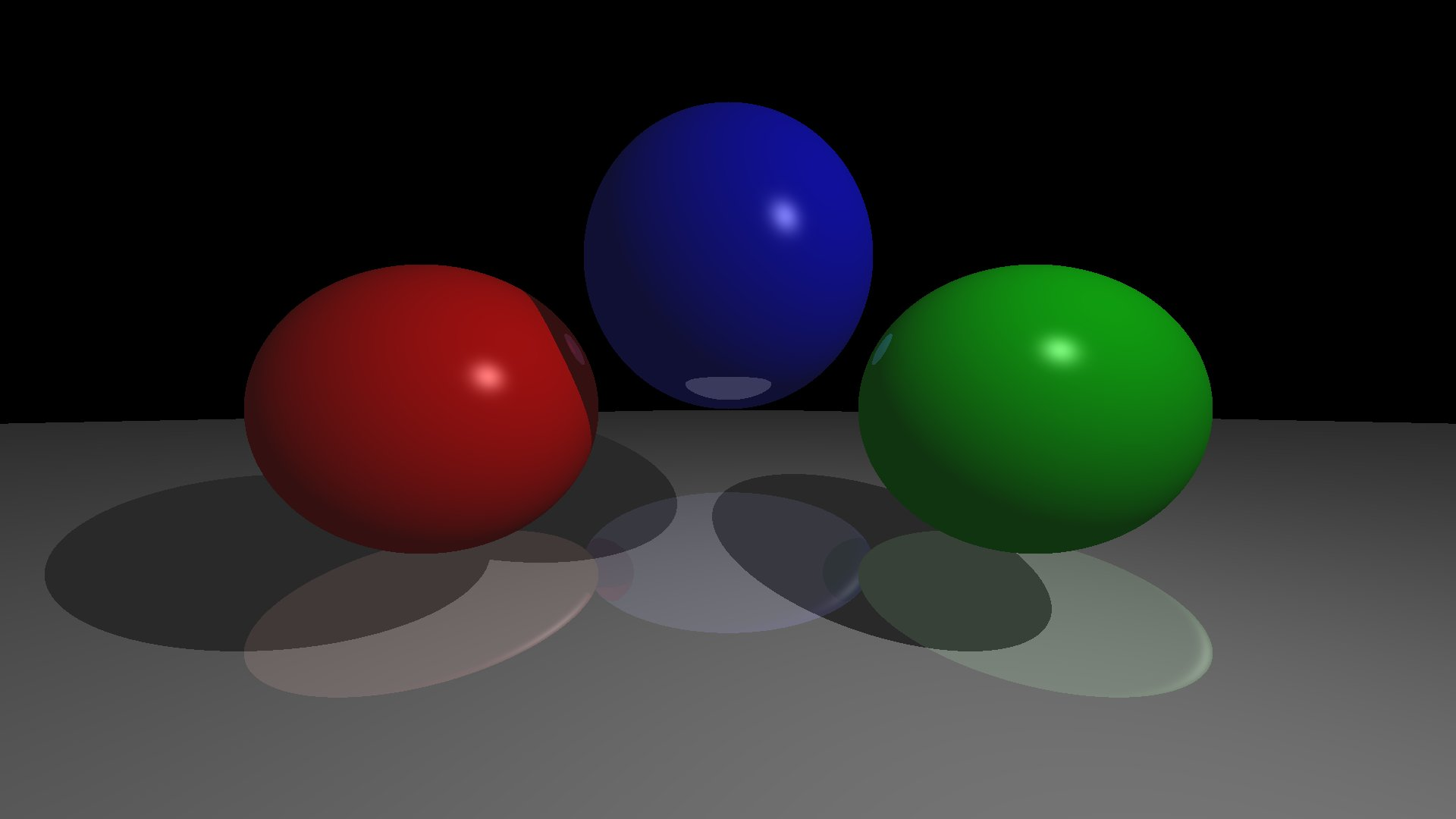Figure 7: Reflections on plane and spheres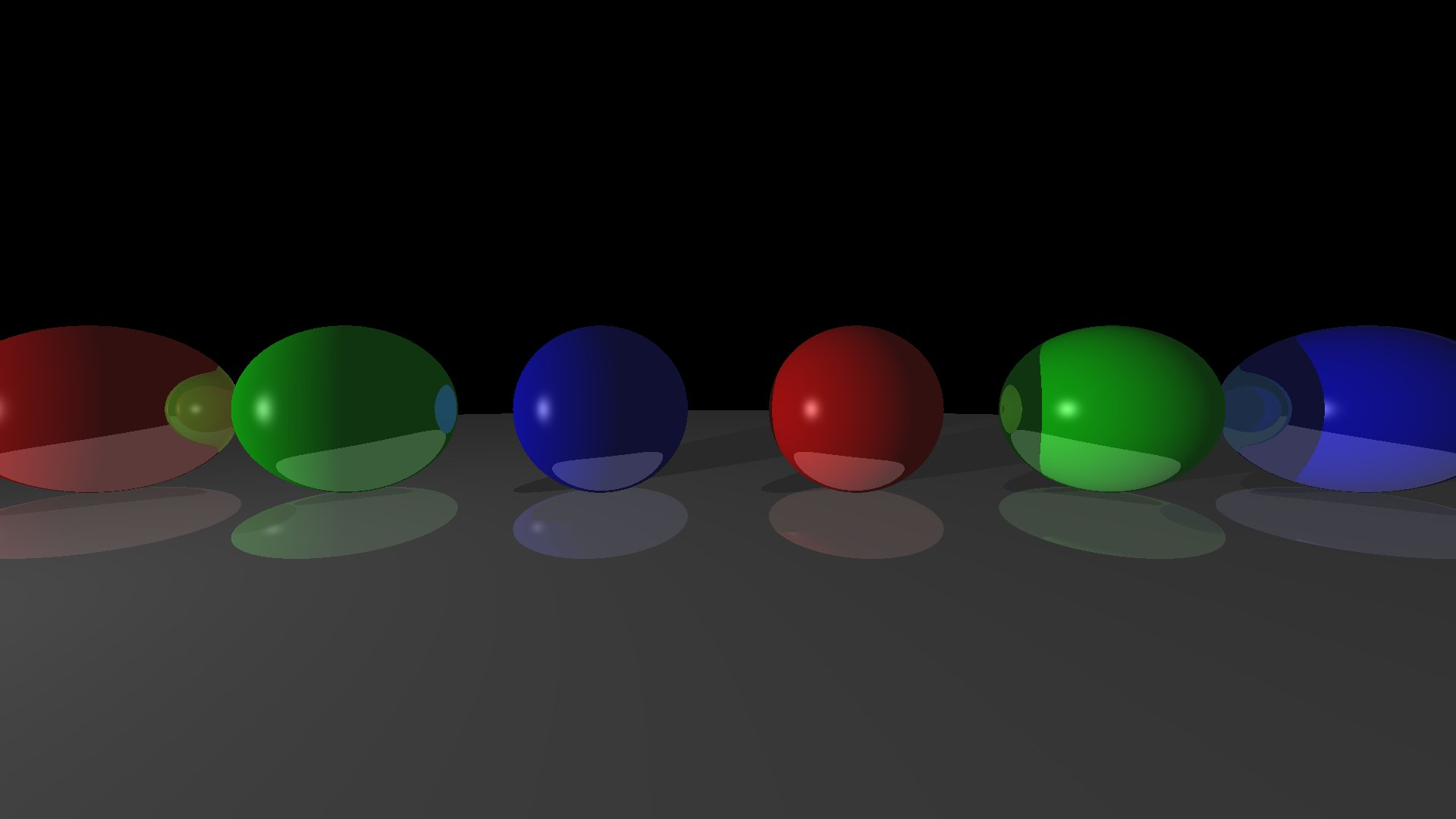Figure 8: Another example of reflections